# R语言中广义线性模型(GLM)中的分布和连接函数分析

``````x = c(1,2,3,4,5)
y = c(1,2,4,2,6)
base = data.frame(x,y)``````

``````regNId = glm(y~x,family=gaussian(link="identity"),data=base)

``````
plot(x,y,pch=19)
abline(regNId,col=darkcols)
abline(regPId,col=darkcols)
abline(regGId,col=darkcols)
abline(regIGId,col=darkcols)
abline(regTwId,lty=2)``````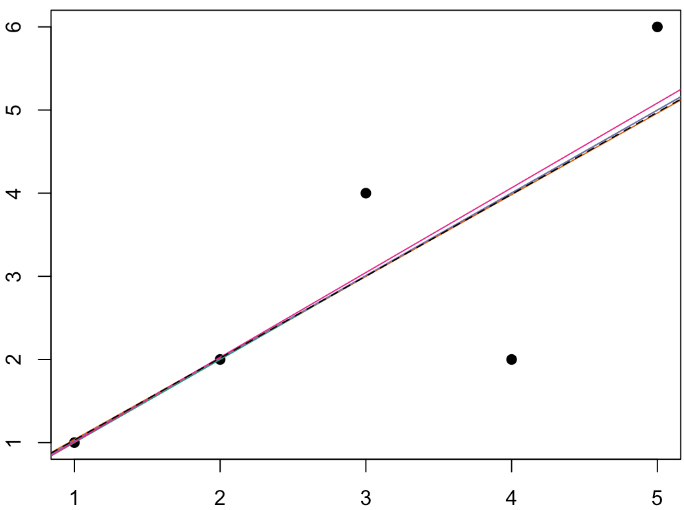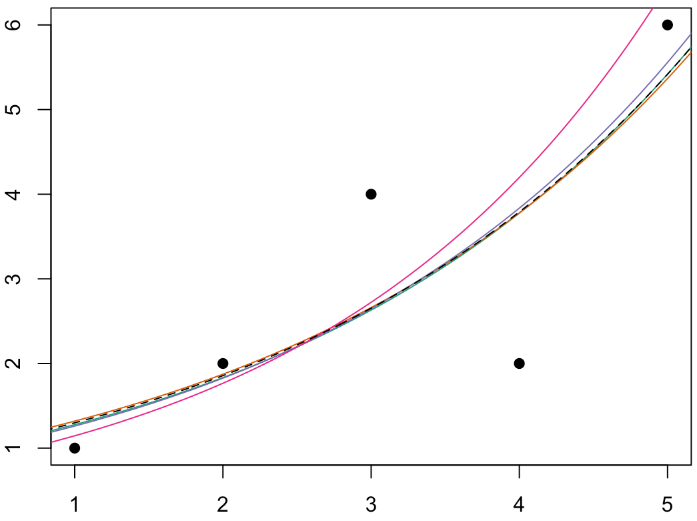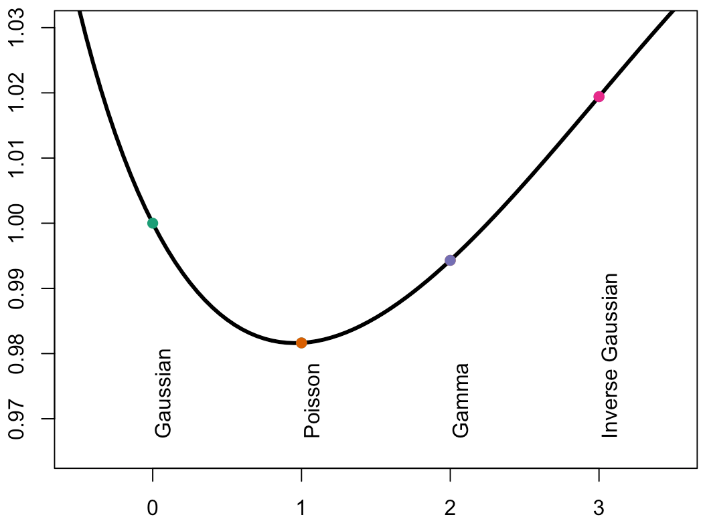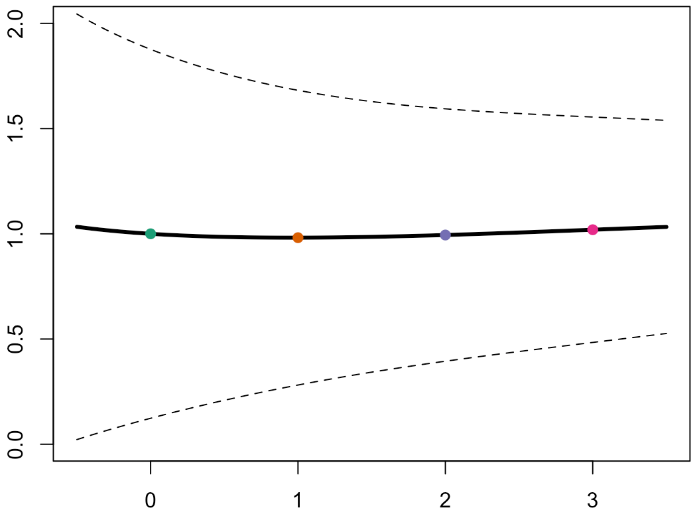``````

plot(Vgamma,Verreur,type="l",lwd=3,ylim=c(-.1,.04),xlab="power",ylab="error")
abline(h=0,lty=2)``````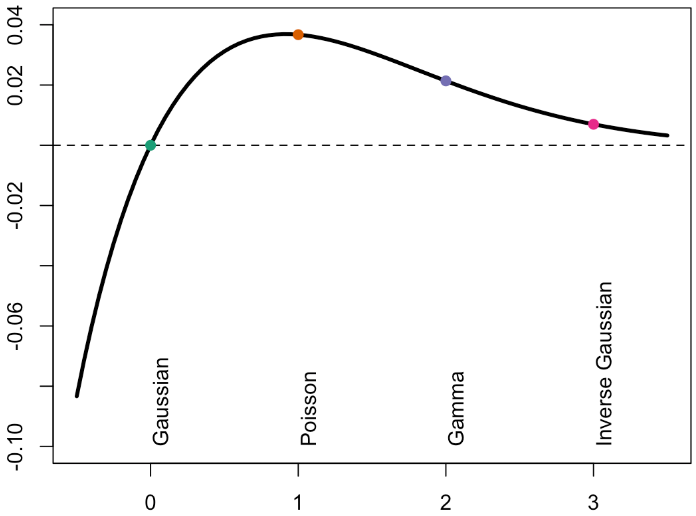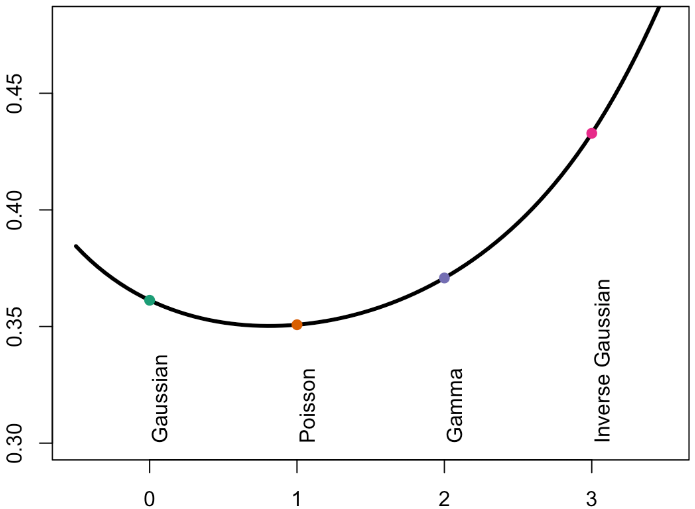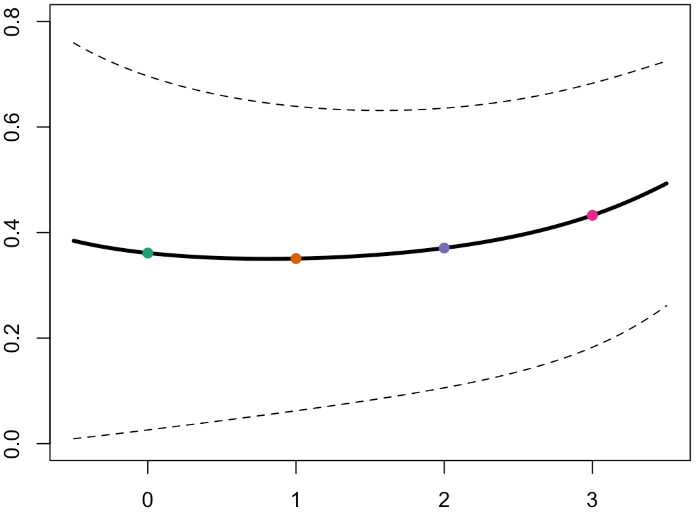​

``````
plot(Vgamma,Verreur,type="l",lwd=3,ylim=c(.001,.32),xlab="power",ylab="error")``````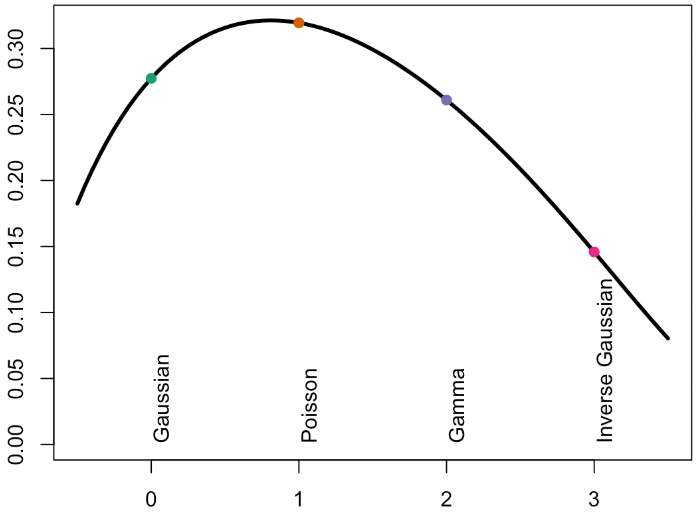Kaizong Ye拓端研究室（TRL）的研究员。

​非常感谢您阅读本文，如需帮助请联系我们！QQ在线咨询

15121130882

0571-63341498

## 关注有关新文章的微信公众号

This will close in 0 seconds